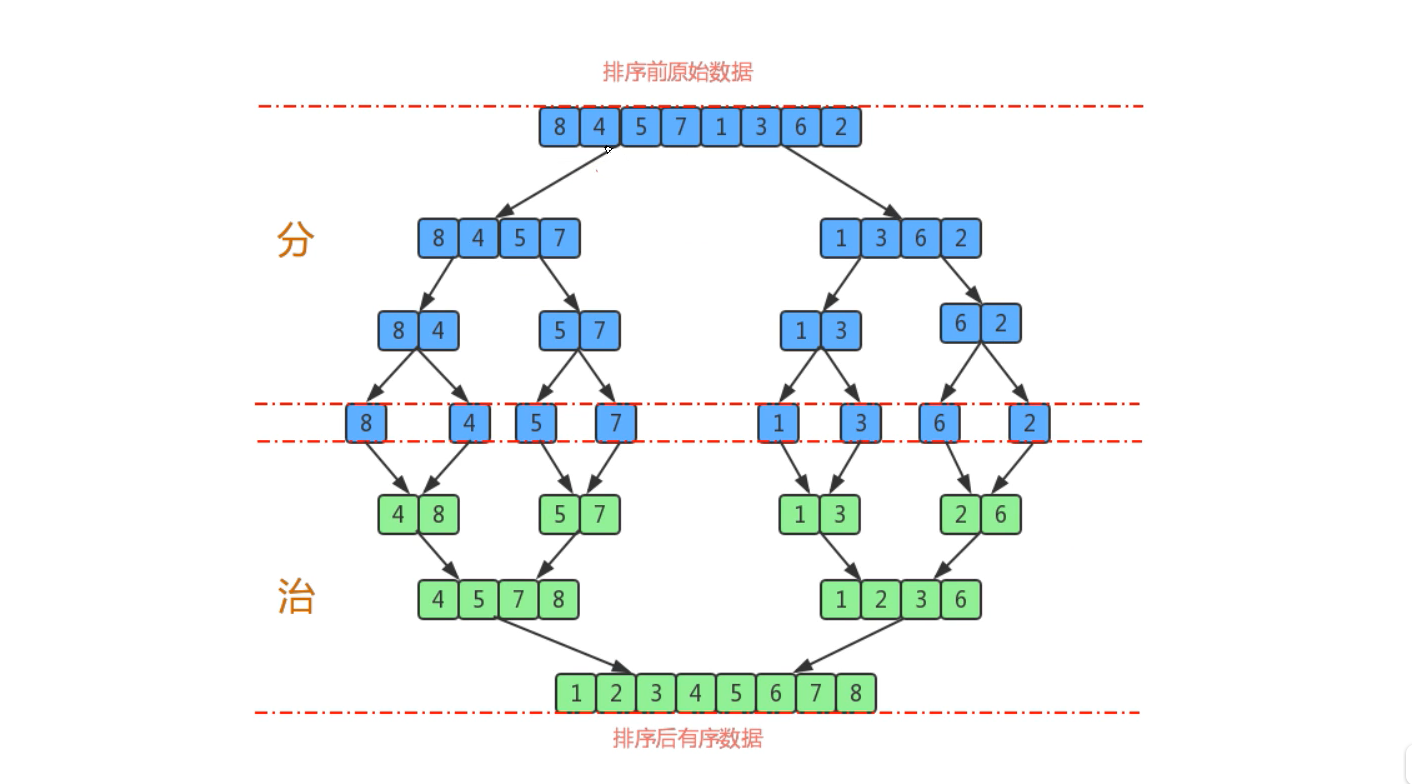归并排序(Merge Sort)

xiaohai 2021-06-16 10:25:02 1324人围观 标签： 算法

3.5.1、需求

• 排序前：{8, 4, 5, 7, 1, 3, 6, 2}
• 排序后：{1, 2, 3, 4, 5, 6, 7, 8}

3.5.2、排序原理

• 尽可能的将一组数据拆分成两个元素相等的子组，并对每个子组继续进行拆分，知道拆分后的每个子组的元素个数是1为止
• 将相邻的两个子组进行合并成一个有序的大组
• 不断的重复步骤2，知道最终只有一个组为止3.5.3、API设计

private static void sort(Comparable[] a,int lo,int hi)：对数组a中从索引lo到索引hi之间的元素进行排序
private static void merge(Compareble[] a,int lo,init mid,int hi)：从索引lo到索引mid为一个子组，从索引mid+1到索引hi为另一个子组，把数组a的这个两个子组数据合成一个有序的大组（从索引lo到索引hi）
private static boolean grater(Comparable v,Comparable w)：判断v是否大于w
private static void exch(Comparable[] a,int i,int j)：交换a数组中，索引i和索引j处的值

3.5.4、代码实现

package cn.test.algorithm.sort;

public class Merge {
private static Comparable[] assist;

/**
* 对数组元素进行排序
*
* @param a
*/
public static void sort(Comparable[] a) {
//初始化辅助数组
assist = new Comparable[a.length];
//定义一个lo变量，和hi变量，分别记录数组中最小的索引和最大的索引
int lo = 0;
int hi = a.length - 1;
//调用sort重载方法完成数组a中，从索引lo到索引hi的元素进行排序
sort(a, lo, hi);
}

//对lo到hi的元素进行排序
private static void sort(Comparable[] a, int lo, int hi) {
//安全性校验
if (hi <= lo) {
return;
}

//对lo到hi的数据进行分组
int mid = lo + (hi - lo) / 2;//mid作为中间值，分割数组

//分组后对每组数据进行排序
sort(a, lo, mid);
sort(a, mid + 1, hi);

//排序后对数据进行归并
merge(a, lo, mid, hi);
}

//合并数组并排序
private static void merge(Comparable[] a, int lo, int mid, int hi) {
//这里需要三个指针
int i = lo;//辅助数组的开始位置指针
int p1 = lo;//左边数组的开始位置指针
int p2 = mid + 1;//右边数组的开始位置指针

//对左右数组的数据进行比较，并插入到辅助数组中
while (p1 <= mid && p2 <= hi) {
if (greater(a[p1], a[p2])) {
assist[i++] = a[p2++];
} else {
assist[i++] = a[p1++];
}
}

//需要判断左边数组是否都移动完了
while (p1 <= mid) {
assist[i++] = a[p1++];
}

//需要判断右边数组是否都移动完了
if (p2 <= hi) {
assist[i++] = a[p2++];
}

//需要将辅助数组的数据复制到原数组中
for (int index = lo; index <= hi; index++) {
a[index] = assist[index];
}

}

/**
* 比较v元素是否大于w元素
*
* @return
*/
private static boolean greater(Comparable v, Comparable w) {
return v.compareTo(w) > 0;
}

/**
* 交换i和j处的元素值
*
* @param a
* @param i
* @param j
*/
private static void exch(Comparable[] a, int i, int j) {
Comparable temp;
temp = a[i];
a[i] = a[j];
a[j] = temp;
}
}

package cn.test.algorithm.test;

import cn.test.algorithm.sort.Merge;

import java.util.Arrays;

public class MergeTest {
public static void main(String[] args) {
Integer[] arr = {8, 4, 5, 7, 1, 3, 6, 2};
Merge.sort(arr);

System.out.println(Arrays.toString(arr));
}
}

[1, 2, 3, 4, 5, 6, 7, 8]